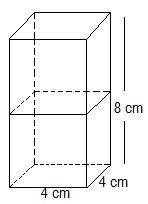# 2 cubes each of volume 64 cm3 are joined end to end. Find the surface area of the resulting cuboid.Volume of cube=64cm= (side)3

(Side)= 64

Side = (64cm31/3

= (2*2*2*2*2*2 cm3)1/3

= 2*2

= 4 cm

Hence,

Length = 8 cm

Height = 4 cm

Surface area of cuboid=2(lb+bh+hl)

=2(8*4+4*4+4*8)

=2(32+16+32)

=2(80)

=160cm2